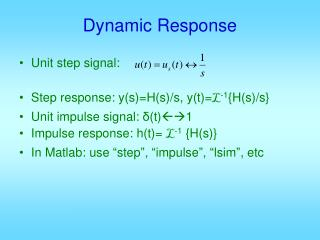Download PresentationDynamic Response

Loading in 2 Seconds...

# Dynamic Response - PowerPoint PPT Presentation

Unit step signal: Step response: y(s)=H(s)/s, y(t)= L -1 {H(s)/s} Unit impulse signal: δ (t) 1 Impulse response: h(t)= L -1 {H(s)} In Matlab: use “step”, “impulse”, “lsim”, etc. Dynamic Response. Defined based on unit step response Defined for closed-loop systemI am the owner, or an agent authorized to act on behalf of the owner, of the copyrighted work described.
Download Presentation## Dynamic Response

An Image/Link below is provided (as is) to download presentation

Download Policy: Content on the Website is provided to you AS IS for your information and personal use and may not be sold / licensed / shared on other websites without getting consent from its author.While downloading, if for some reason you are not able to download a presentation, the publisher may have deleted the file from their server.

- - - - - - - - - - - - - - - - - - - - - - - - - - E N D - - - - - - - - - - - - - - - - - - - - - - - - - -
Presentation Transcript
1. Unit step signal: Step response: y(s)=H(s)/s, y(t)=L-1{H(s)/s} Unit impulse signal: δ(t)1 Impulse response: h(t)= L-1 {H(s)} In Matlab: use “step”, “impulse”, “lsim”, etc Dynamic Response

2. Defined based on unit step response • Defined for closed-loop system • Steady-state value yss • Steady-state error ess • Settling time ts = time when y(t) last enters a tolerance band Time domain response specifications

3. By final value theorem In MATLAB: num = [ .. .. .. .. ] b0 = num(length(num)), or num(end) a0 = den(length(den)), or den(end) yss=b0/a0

4. If numerical values of y(t) available, abs(y – yss) < tol means inside band abs(y – yss) ≥ tol not inside e.g. t_out = t(abs(y – yss) ≥ tol) contains all those time points when y is not inside the band. Therefore, the last value in t_out will be the settling time. ts=t_out(end)

5. Peak time tp = time when y(t) reaches its maximum value. Peak value ymax =y(tp) Hence: ymax = max(y); tp = t(y = ymax); Overshoot: OS = ymax - yss Percentage overshoot:

6. If ymaxis reached as t→∞, there is no peak time and there is no overshoot. Delay time td = the time when y(t) first reaches 50% of yss • Not frequently used • Some people use a percentage different from 50% t50=t(y<=0.5*yss); td=t50(end)

7. Rise time tr = the time it takes for y(t) to go from 0.1yssto 0.9yss for the first time. • Rise time captures how fast a system responds to changes in a reference input • td,tphas similar effect

8. If t50 = t(y >= 0.5·yss), this contains all time points when y(t) is ≥ 50% of yss so the first such point is td. td=t50(1); Similarly, t10 = t(y >= 0.1*yss) & t90 = t(y >= 0.9*yss) can be used to find tr. tr=t90(1)-t10(1)

9. 90%yss tr≈0.45 10%yss ts ts tp≈0.9sec td≈0.35

10. ±5% ts=0.45 yss=1 ess=0 O.S.=0 Mp=0 tp=∞ td≈0.2 tr≈0.35

11. tp=0.35 O.S.=0.4 Mp=40% yss=1 es=0 ts≈0.92 td≈0.2 tr≈0.1

12. Steady-state tracking & sys. types • Unity feedback control: plant + e r(s) y(s) C(s) G(s) - + e r(s) Go.l.(s) y(s) -

13. r(t) t

14. r(t) 0 t

15. ref. input sys. type

16. Example of tank + C H -

17. e.g. Kps+KI s ωn2 s(s+2ξ ωn) 1 Ts+1 + e r(s) - r(s) +

18. example e(s) y(s) r(s) G(s)